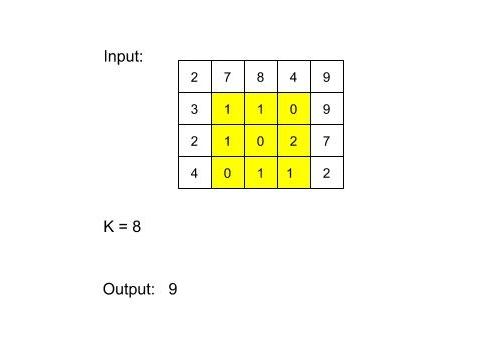# Square Submatrix with sum less than or equal to K

Posted: 12 Jan, 2021
Difficulty: Moderate## PROBLEM STATEMENT

#### For example :#### If there is no square sub-matrix with sum less than or equal to K, then return 0.

##### Input Format
``````The first line of input contains an integer 'T' representing the number of test cases. Then the test cases follow.

The first line of each test case contains three single-spaced integers N, M and K, representing the number of rows, number of columns and the given integer, respectively.

The next N line contains M single-spaced elements.
``````
##### Output Format:
``````For each test case, print the size of the largest sub-matrix with sum less than or equal to K.

The output for each test case is in a separate line.
``````
##### Note:
``````You do not need to print anything, it has already been taken care of. Just implement the given function.
``````
##### Constraints:
``````1 <= T <= 5
1 <= N, M <= 100
0 <= K <= 10^5,
0 <= data <= 10^5,

where ‘T’ is the number of test cases,  ‘N’ and ‘M’ are the numbers of rows and columns respectively and ‘data’ is the value of the elements of the matrix.
``````Approach 1

In this approach, we will find all possible square sub-matrices and check whether it has sum less than or equal to K or not.

We will define a square sub-matrix using 3 variables (R, C, L). (R, C) denotes the top-left corner of the sub-matrix and L denotes the number of rows/column in the square sub-matrix. So, (R+L-1, C+L-1) will be the bottom-right corner of the sub-matrix.

For (R: 0 to N-1){

For (C: 0 to M-1){

For(L: 1 to Min((N-R),(M-C) )){

Find the sum of sub-matrix (R, C, R+L-1, C+L-1)

}

}

}

For finding the sum of a sub-matrix, we will iterate on the elements of the sub-matrix using two loops.

In this approach, we will use the prefix sum, so that we don’t have to iterate on the elements of the sub-matrix for finding the sum of a sub-matrix.

Let pref[][] store the prefix sum of the given matrix.

pref[i][j] is the sum of the sub-matrix whose top-left corner is at (0, 0) and bottom right corner is at (i, j).

For finding the prefix matrix, first, we will do the row-wise sum and then we will do the column-wise sum.

For example:

Sum of a sub-matrix (R1, C1, R2, C2) {where (R1, C1) denotes the top-left corner of the sub-matrix and (R2, C2) denotes the bottom-right corner of the sub-matrix} is:

SUM = pref[R2][C2] - pref[R2][C1-1] - pref[R1-1][C2] + pref[R1-1][C1-1].

If S is the sum of a square submatrix whose top-left corner is (R, C) and contains L number of rows/columns, then the sum of the square submatrix with same top-left corner, containing (L+1) number of rows/columns is always greater than or equal to S (since the matrix contains non-negative integers).

It means using binary search we can find the largest square submatrix whose top-left corner is (R, C).

We will iterate on the top-left index of the sub-matrix and, using binary search, we will find the largest submatrix from this index.

If (R, C) denotes the top-left corner of the sub-matrix, then we have to find the largest L such that the sum of the submatrix  (R, C, R+L-1, C+L-1) is less than or equal to K.

For (R: 0 to N-1){

For (C: 0 to M-1){

BinarySearch(L: 1 to Min((N-R),(M-C) )

}

}

For finding the sum of a sub-matrix, we will use the prefix sum.